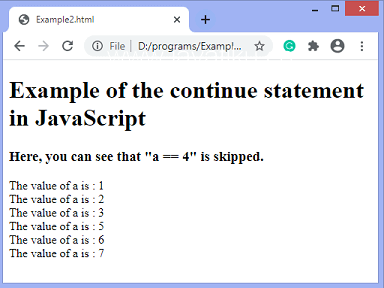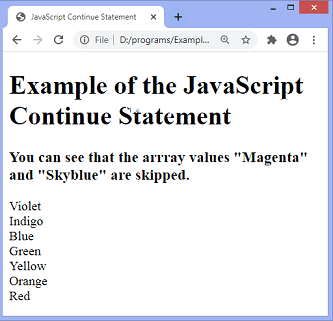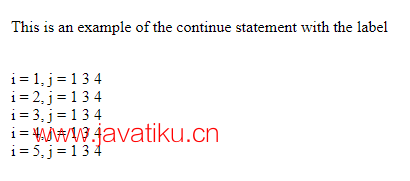# JavaScript教程-JavaScript continue 语句### 语法

``continue;``

``continue[label]; // 使用标签引用``

### 示例1

``````<!DOCTYPE html>
<html>

<body>
<h1> JavaScript中continue语句的示例 </h1>
<h3> 在这里，您可以看到 "a == 4" 被跳过。 </h3>
<p id="para">
</p>
<script>
var res = "";
var a;
for (a = 1; a <= 7; a++) {
if (a == 4) {
continue;
}
res += "a的值为: " + a + "<br>";
}
document.getElementById("para").innerHTML = res;
</script>
</body>

</html>``````### 示例2

``````<!DOCTYPE html>
<html>

<title> JavaScript Continue 语句的示例 </title>

<body>
<h1> JavaScript Continue 语句的示例 </h1>
<h3> 您可以看到数组值 "Magenta" 和 "Skyblue" 被跳过。 </h3>
<script>
var rainbow = ["Violet", "Indigo", "Magenta", "Blue", "Skyblue", "Green", "Yellow", "Orange", "Red"];
var i = 0;
var res = "";
while (i < rainbow.length){
if (rainbow[i] == "Magenta" || rainbow[i] == "Skyblue") {
i++;
continue;
}
res = "";
res += rainbow[i] + "<br>";
i++;
document.write(res);
}
</script>
</body>

</html>``````### 示例3

``````<!DOCTYPE html>
<html>

<body>
<p> 这是带有标签的continue语句示例 </p>
<p id="para"> </p>
<script>
var res = "";
var i, j;

label1: // 这个循环被标记为"label1"
for (i = 1; i <= 5; i++) {
res += "<br>" + "i = " + i + ", j = ";

label2: // 这个循环被标记为"label2"
for (j = 1; j <= 4; j++) {
if (j == 2) {
continue label2;
}
document.getElementById("para").innerHTML = res += j + " ";
}
}
</script>

</body>

</html>``````